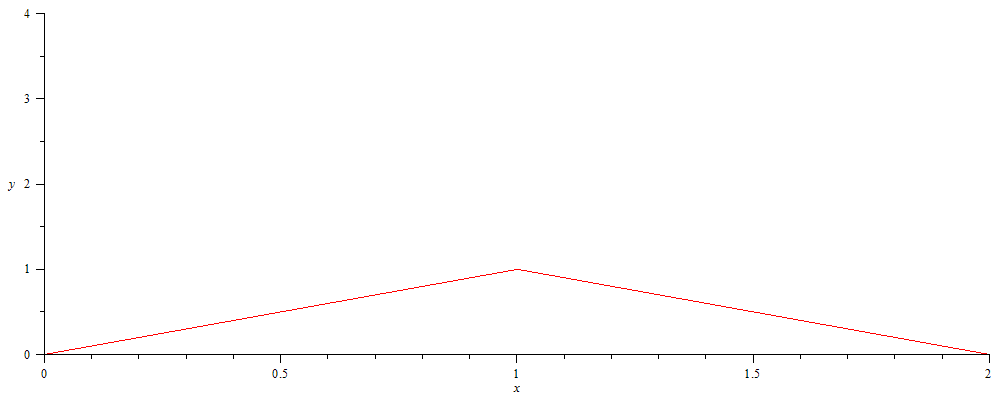# Insult and injury

Scene: Advanced Calculus II class.  I am finishing the proof of the construction Hardy’s zig-zagging continuous everywhere, differentiable nowhere function:$displaystyle h(x) = sum_{n=0}^infty frac{2}{pi} bigg( frac{3}{4} bigg)^n bigg| arcsin bigg( sin bigg( frac{4^n pi}{2} x bigg) bigg) bigg|$Me: …and so we’ve shown$displaystyle lim_{m to infty} bigg| frac{h(x_m) - h(x_0)}{x_m - x_0} bigg| = + infty$, which proves the function is not differentiable at$x_0$.

Student A:  Can you remind me why?

Me: Sure.  Essentially, this argument shows that the difference quotient limit — the derivative — is blowing up at every point simultaneously.

Student B:  Huh.  You’re like the Michael Bay of calculus.

Me: Get the hell out of my classroom.

This entry was posted in academify, nerdify. Bookmark the permalink.